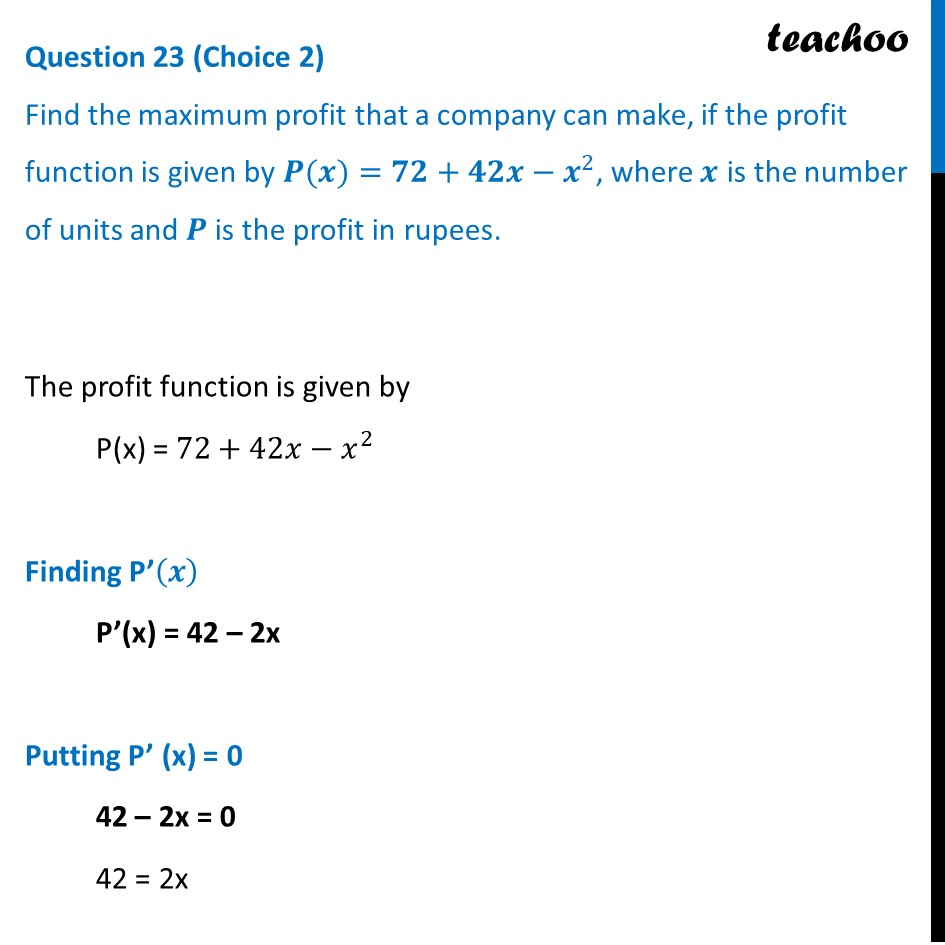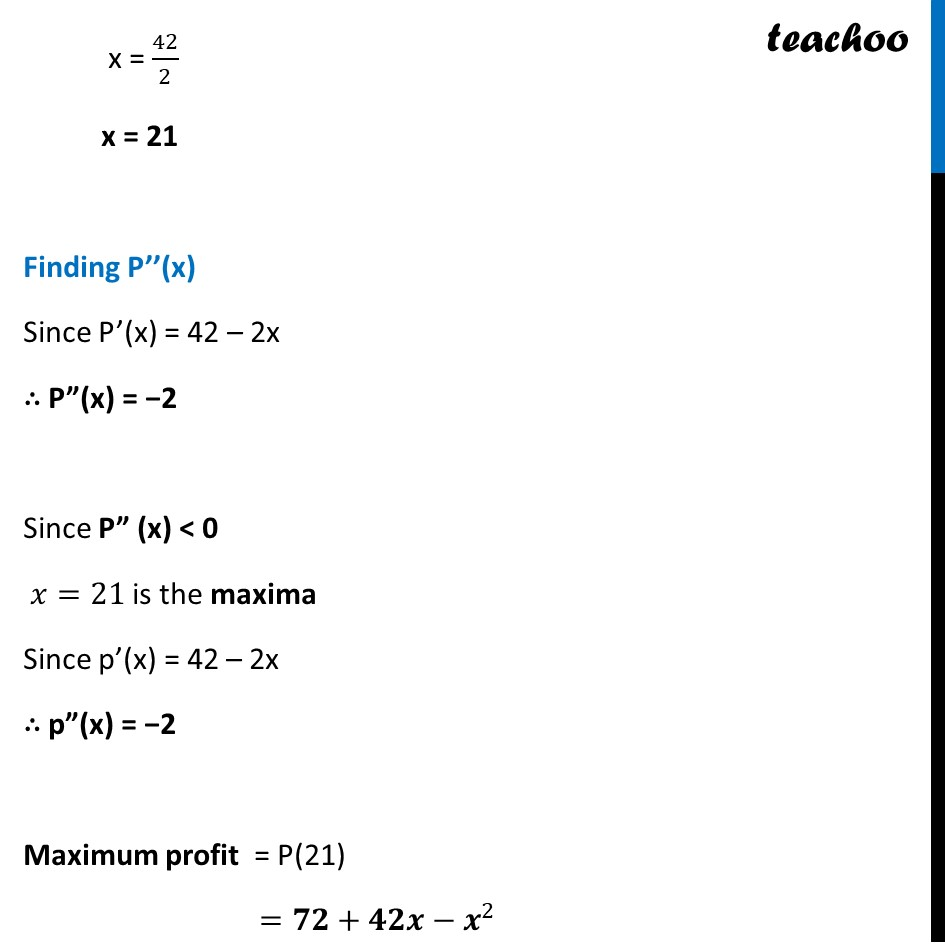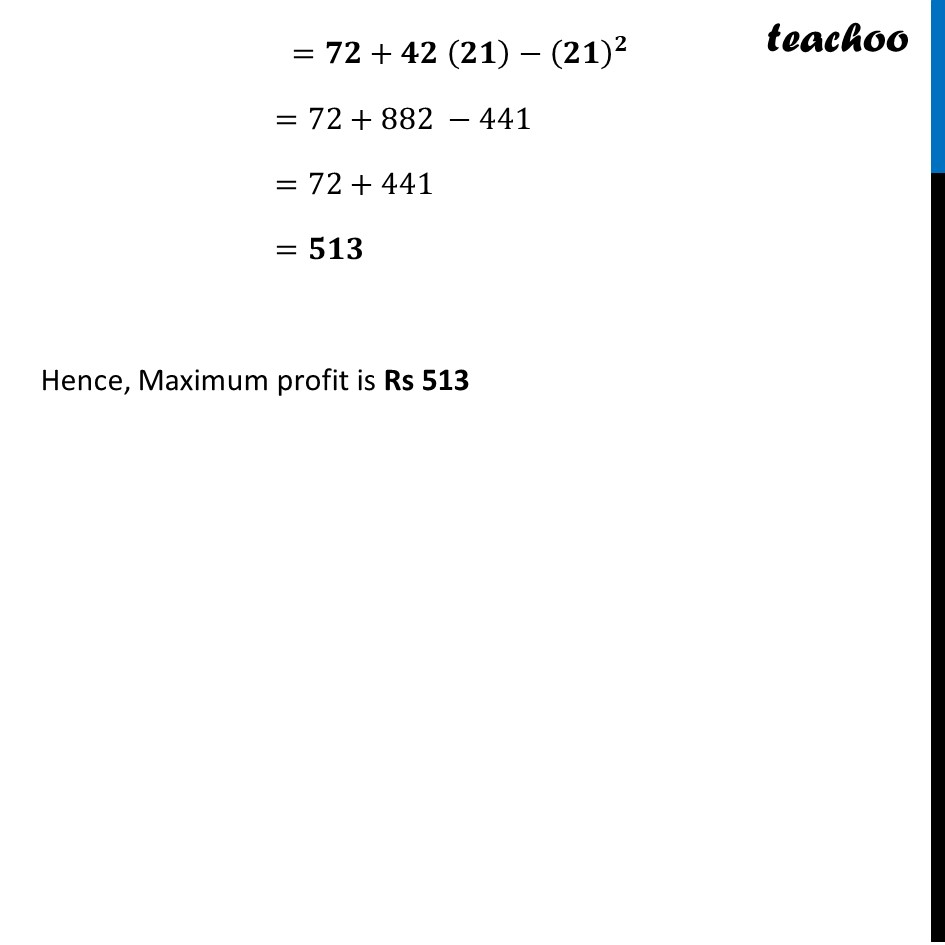CBSE Class 12 Sample Paper for 2024 Boards

Class 12
Solutions of Sample Papers and Past Year Papers - for Class 12 Boards

## Find the maximum profit that a company can make, if the profit function is given by P(x)=72+42x-x^2, where x is the number of units and P is the profit in rupees.

This question is similar to Ex-6.5, 6 Chapter 6 Class 12Learn in your speed, with individual attention - Teachoo Maths 1-on-1 Class

### Transcript

The profit function is given by P(x) = 72+42𝑥−𝑥^2 Finding P’(𝒙) P’(x) = 42 – 2x Putting P’ (x) = 0 42 – 2x = 0 42 = 2x x = 42/2 x = 21 Finding P’’(x) Since P’(x) = 42 – 2x ∴ P”(x) = −2 Since P” (x) < 0 𝑥=21 is the maxima Since p’(x) = 42 – 2x ∴ p”(x) = −2 Maximum profit = P(21) =𝟕𝟐+𝟒𝟐𝒙−𝒙^2 =𝟕𝟐+𝟒𝟐 (𝟐𝟏)−(𝟐𝟏)^𝟐 =72+882 −441 =72+441 =𝟓𝟏𝟑 Hence, Maximum profit is Rs 513